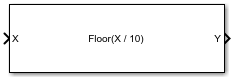Divide by Constant and Round

Divide input by a constant and round to integer

• Library:
• Fixed-Point Designer

•Description

The Divide by Constant and Round block outputs the result of dividing the input by a constant and rounds the result to an integer using the specified rounding method.

The Divide by Constant and Round block uses an algorithm that is functionally similar to the Granlund-Montgomery-Warren Method. The division operation is computed via a multiplication by inverse, which generally results in better performance on embedded systems.

Ports

Input

expand all

Dividend, specified as a scalar, vector, matrix, or N-D array.

Divide by Constant and Round does not support data types with word length greater than 128.

Data Types: single | double | int8 | int16 | int32 | uint8 | uint16 | uint32 | Boolean | fixed point
Complex Number Support: Yes

Output

expand all

Result of division and round operation, returned as a scalar, vector, matrix, or N-D array.

Data Types: single | double | int8 | int16 | int32 | uint8 | uint16 | uint32 | Boolean | fixed point

Parameters

expand all

Divisor, specified as a positive, real-valued, finite scalar.

Programmatic Use

 Block Parameter: Denominator Type: character vector Values: MATLAB® expression that evaluates to a positive, real-valued, finite fixed point or numeric value Default: '10'

Data Types: single | double | int8 | int16 | int32 | int64 | uint8 | uint16 | uint32 | uint64 | Boolean | fixed point

Rounding method to use, specified as one of these values:

• Floor — Round to nearest integer in the direction of negative infinity.

• Ceiling — Round to nearest integer in the direction of positive infinity.

• Nearest — Round to the nearest integer. Ties are rounded to the nearest integer in the direction of positive infinity.

• Zero — Round to the nearest integer in the direction of zero.

• Convergent — Round to the nearest integer. Ties are rounded to the nearest even integer.

Programmatic Use

 Block Parameter: RndMeth Type: character vector Values: 'Floor' | 'Ceiling' | 'Nearest' | 'Zero' | 'Convergent' Default: 'Floor'

Extended Capabilities